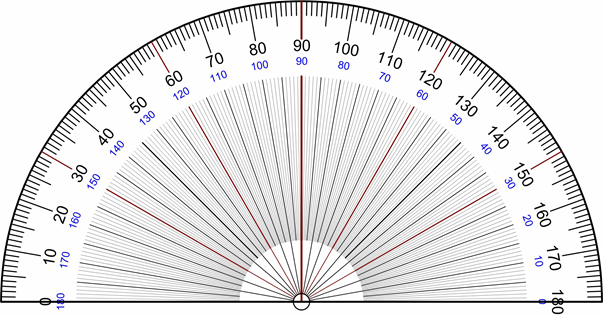# Shapes and Angles

## Objective

Sketch given angle measures and verify with a protractor.

## Common Core Standards

### Core Standards

?

• 4.MD.C.5 — Recognize angles as geometric shapes that are formed wherever two rays share a common endpoint, and understand concepts of angle measurement:

• 4.MD.C.6 — Measure angles in whole-number degrees using a protractor. Sketch angles of specified measure.

## Criteria for Success

?

1. Sketch angles of various measures using a protractor by drawing a straight line for the base ray, aligning that ray to the baseline of the protractor, drawing a point at the desired angle measure, aligning the vertex of the angle and the point just drawn, and drawing the second ray (MP.5, MP.6).
2. Check angle measures by using the protractor (MP.5, MP.6).
3. Understand that various angles can be sketched with the same angle measure.

#### Fishtank Plus

• Problem Set
• Student Handout Editor
• Vocabulary Package

?

### Problem 1

Ms. Kosowsky measured an angle that is 60°. Show what her angle looked like on the protractor below:#### References

Wikimedia Commons Photo: Protractor Rapporteur Degrees V3

Protractor Rapporteur Degrees V3 is made available on Wikimedia Commons under the CC0 1.0 license. Accessed Feb. 20, 2018, 9:35 a.m..

### Problem 2

Sketch angles that have each of the following angle measures:

1. 80°
2. 133°

#### References

EngageNY Mathematics Grade 4 Mathematics > Module 4 > Topic B > Lesson 7Concept Development Problem 4

Grade 4 Mathematics > Module 4 > Topic B > Lesson 7 of the New York State Common Core Mathematics Curriculum from EngageNY and Great Minds. © 2015 Great Minds. Licensed by EngageNY of the New York State Education Department under the CC BY-NC-SA 3.0 US license. Accessed Dec. 2, 2016, 5:15 p.m..

Modified by Fishtank Learning, Inc.

## Problem Set & Homework

### Discussion of Problem Set

• What was different about #2e and #2f compared to the previous angles you drew? How did you draw angles of these measures?
• What does your angle in #3 look like? Was it easier or more difficult to draw this angle?
• What does your angle in #4 look like? Is there more than one correct answer? What must be true about the angle measure, though?
• Why is it important to be precise when drawing angles? Tell your partner how you can be precise when drawing angles.
• Why do we verify our sketches with a protractor?

?

Draw angles that measure the given number of degrees. Draw an arc to indicate the angle that was measured.

a.   55°

b.   130°

c.    78°

d.   104°

#### Mastery Response